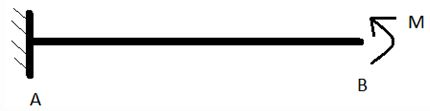Courses

# Test: The Double Integration Method

## 15 Questions MCQ Test | Test: The Double Integration Method

Description
This mock test of Test: The Double Integration Method for Civil Engineering (CE) helps you for every Civil Engineering (CE) entrance exam. This contains 15 Multiple Choice Questions for Civil Engineering (CE) Test: The Double Integration Method (mcq) to study with solutions a complete question bank. The solved questions answers in this Test: The Double Integration Method quiz give you a good mix of easy questions and tough questions. Civil Engineering (CE) students definitely take this Test: The Double Integration Method exercise for a better result in the exam. You can find other Test: The Double Integration Method extra questions, long questions & short questions for Civil Engineering (CE) on EduRev as well by searching above.
QUESTION: 1

### Which of the following is correct boundary condition for a beam supported by pin at both ends?

Solution:

Explanation: Since there will always be a vertical support reaction, displacement at both ends will be zero.

QUESTION: 2

### Which of the following is false for deflection of a point nearby a fixed support?

Solution:

Explanation: Due to presence of vertical reaction and moment, there won’t be any displacement and slope will be zero.

QUESTION: 3

### The double integration method to calculate slope of deflected beam is applicable only when:-

Solution:

Explanation: During deriving the results, we have assumed that slope is zero once.

QUESTION: 4

Which out of the following is true for x axis:-

Solution:

Explanation: During deriving, we have assumed x axis to be parallel to undeflected beam.

QUESTION: 5

Where does origin lies?

Solution:

Explanation: Origin is assumed to be at left of beam and rightward is positive.

QUESTION: 6

Positive value of slope is clockwise.
State whether the above statement is true or false.

Solution:

Explanation: Positive value of slope is counter clockwise.

QUESTION: 7

Following is a cantilever beam and its length is Z.
A moment M is applied at the end B.
E and I are given.What is the degree of static indeterminacy of this question?

Solution:

Explanation: This has 3 unknown reactions and three equations which make it statically determinate.

QUESTION: 8

Following is a cantilever beam and its length is Z.
A moment M is applied at the end B.
E and I are given.What will be value of double differentiation of deflection in y direction wrt distance from point A at point A?

Solution:

Explanation: Since moment M is acting counterclockwise, moment at A will be clockwise and will be equal to M.

QUESTION: 9

Following is a cantilever beam and its length is Z.
A moment M is applied at the end B.
E and I are given.How many boundary conditions will be required to solve this question?

Solution:

Explanation: Since double differentiation of deflection in y direction wrt distance from point A is independent of distance from point A, there will be only two unknown constants which would require 2 equations/boundary conditions.

QUESTION: 10

Following is a cantilever beam and its length is Z.
A moment M is applied at the end B.
E and I are given.What will be the value of differentiation of deflection in y direction wrt distance from point A at point A?

Solution:

Explanation: Value will be zero as slope is zero due to fixed support.

QUESTION: 11

Following is a cantilever beam and its length is Z.
A moment M is applied at the end B.
E and I are given.Value of deflection in y direction at point B will be zero.
State whether the above statement is true or false.

Solution:

Explanation: We can’t predict deflection in y direction at point B as it is a free end

QUESTION: 12

Following is a cantilever beam and its length is Z.
A moment M is applied at the end B.
E and I are given.What will be the value of first obtained constant?

Solution:

Explanation: By replacing value of double differentiation of deflection in y direction wrt distance from point A at point A will give this result.

QUESTION: 13

Following is a cantilever beam and its length is Z.
A moment M is applied at the end B.
E and I are given.What will be the value of second obtained constant?

Solution:

Explanation: By replacing value of differentiation of deflection in y direction wrt distance from point A at point A will give this result.

QUESTION: 14

Following is a cantilever beam and its length is Z.
A moment M is applied at the end B.
E and I are given.What is slope at point B?

Solution:

Explanation: By solving after putting value of first constant will give this MZ/EI.

QUESTION: 15

Following is a cantilever beam and its length is Z.
A moment M is applied at the end B.
E and I are given.What is deflection in y direction at point B?

Solution: